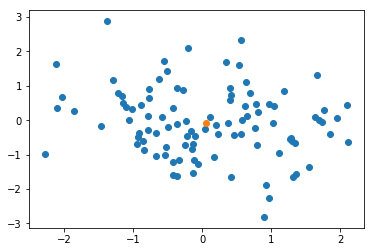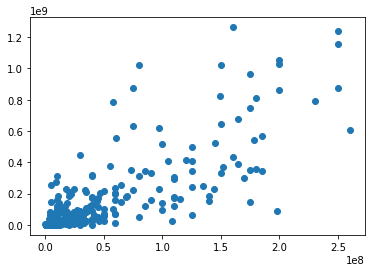Do movies that cost more make more money?

In this unit of the class we are going to consider ways to measure relationships between variables, such as budget and revenue.

This cell is a "markdown" cell. It displays formatted text in italics bold monospace and $m^at_h$.

In :
## You will often see import statements with
##  aliases: import numpy as np
## I can't see any reason to do this other than the
##  fact that lots of people seem to do it.
## Personally, if I have to choose between readability
##  and a few extra keystrokes, I will ALWAYS choose
##  readability, but if you are used to seeing np.,
##  that might be more readable.
## For beginning programming I prefer to keep things
##  simple and direct, but be aware that people may
##  expect you to use aliases, and may judge you.

import numpy
from matplotlib import pyplot

## The pandas library provides tools for working with
##  "data frames": tables of data with named columns
##  each with some type (int, float, date, etc)
import pandas

## This is a notebook command that makes sure plots
##  are displayed and not just returned as objects.
%matplotlib inline

## These are special packages for notebooks that
##  give us interactive controls.
from ipywidgets import interact, interactive, fixed


If we're going to use multiple variables we need ways to represent multiple variables.

If every variable is the same type we can combine them into a single numpy array with more than one axis. For two-dimensional arrays by convention we refer to the first axis as rows and the second as columns.

In :
x = numpy.random.normal(0, 1, size=(100,2))
x.shape

Out:
(100, 2)
In :
# to access a cell in the array we can specify an index for both axes (row, column)
x[1,1]

Out:
-0.025806743956922857
In :
# we can ask for more than one value, here the first five rows of the second column
x[0:5,1]

Out:
array([ 0.28624967, -0.02580674, -0.09846675,  0.43131053,  1.60502716])
In :
# if we want every index from a certain axis, we use :
x[1,:]

Out:
array([-0.23507934, -0.02580674])

I now have a matrix with 100 rows and two columns. I can think of the columns as random variables $X_0$ and $X_1$, and each row as a pair $(X_{i0}, X_{i1})$. (I'm trying to be consistent with notation in my variable subscripts: row, then column.) Visualizing the data as a scatter plot can give us an overview.

I know that there is no connection between the variable in the first column and the variable in the second column "by construction": because I just sampled them all independently from a normal distribution. But in the specific case I have here (rerunning the notebook will almost certainly change this), there seems to be a slight downward trend. If I put the mean of the pairs as a second layer (orange), the quadrant left/below the mean looks sparser than the left/above. I know that's just random, but let's see how it affects our measurement of covariance.

In :
pyplot.scatter(x[:,0], x[:,1]) # the x_0, x_1 pairs in blue
pyplot.scatter(x[:,0].mean(), x[:,1].mean()) # the mean in orange
pyplot.show()In :
# first, let's calculate the variance of the two dimensions.
#  the ddof=1 argument means divide by (n-1) instead of n.
# it's around 1, which is good since these values are from
#  a normal with variance 1, and sample size 100.
x[:,0].var(ddof=1)

Out:
1.0584813638646358
In :
# same with the second column. this one is quite close to 1.0.
x[:,1].var(ddof=1)

Out:
1.0079081230212463

Now let's look at the cov command. It doesn't just give us the covariance, it gives us a full "covariance matrix". You should be able to recognize the two variances as the diagonal of the matrix, with the covariance in the two off-diagonal entries.

col 1 col 2
var(A) cov(A, B)
cov(A,B) var(B)

The covariance here is negative, which matches our visual impression that the trend is slightly downward. We know (by construction) that that's purely a coincidence, but it is a measurably true fact about this sample.

Note that cov uses the $\frac{1}{n-1}$ form for variance, while var by default uses the $\frac{1}{n}$ form.

In :
numpy.cov(x[:,0], x[:,1])

Out:
array([[ 1.05848136, -0.19008835],
[-0.19008835,  1.00790812]])

Now let's look at the Pearson correlation coefficient. Numpy returns it to us in the same matrix format as cov, but it's really just one number.

In :
numpy.corrcoef(x[:,0], x[:,1])

Out:
array([[ 1.        , -0.18403627],
[-0.18403627,  1.        ]])

Where did this number come from? It's exactly the same as the covariance divided by the product of standard deviations.

In :
-0.19008835 / (numpy.sqrt(1.05848136) * numpy.sqrt(1.00790812))

Out:
-0.18403626966432207
In :
inputs = numpy.random.normal(0, 1, size=100)
errors = numpy.random.normal(0, 1, size=100)

def show_linear(scale):
outputs = 1.3 * inputs + 0.2 + scale * errors
pyplot.scatter(inputs, outputs)
pyplot.text(-2, 3, str(numpy.corrcoef(inputs, outputs)[0,1]))
pyplot.show()


This next cell is very cool but inscrutable. We are passing the function show_linear as an argument to the function interact. This behavior rarely shows up in Python, it's much more frequent in Javascript. Since show_linear has one argument, scale, we also pass in a tuple that lists a minimum and maximum value for scale along with a step (0.1).

interact will now create an input element for scale. Since the variable is a number based on its value range, the input will be a slider. If we move the slider we call show_linear with scale set to the value of the slider. As we scale up the noise, the correlation coefficient decreases.

In :
interact(show_linear, scale=(0, 2, 0.1))

Out:
<function __main__.show_linear(scale)>

Now let's consider our original question: Do movies with larger budgets have more revenue?

First we need to load the data. I found this on Kaggle, but removed all movies before 2016 and movies with a budget or revenue less than \$100,000. The pandas library makes it easy to load this file: In : movies = pandas.read_csv("movies.csv")  In : movies.describe()  Out: budget id popularity revenue runtime vote_average vote_count count 2.960000e+02 296.000000 296.000000 2.960000e+02 296.000000 296.000000 296.000000 mean 4.639639e+07 316878.361486 20.237346 1.504744e+08 112.016892 6.384797 1158.388514 std 5.647466e+07 73209.405016 34.197320 2.406840e+08 18.190416 0.765532 1531.144387 min 2.000000e+05 14564.000000 0.350207 1.006590e+05 66.000000 4.100000 4.000000 25% 9.000000e+06 291144.750000 8.119095 8.952934e+06 98.000000 5.800000 162.000000 50% 2.200000e+07 330220.000000 12.281325 4.753546e+07 110.000000 6.400000 597.500000 75% 6.000000e+07 368258.250000 17.928330 1.782043e+08 123.000000 6.925000 1570.500000 max 2.600000e+08 443319.000000 294.337037 1.262886e+09 170.000000 8.100000 11444.000000 In : # In a pandas data frame each column becomes an attribute: movies.budget  Out: 0 100000000.0 1 160000000.0 2 230000000.0 3 58000000.0 4 200000000.0 5 250000000.0 6 165000000.0 7 178000000.0 8 3500000.0 9 18000000.0 10 110000000.0 11 180000000.0 12 75000000.0 13 31500000.0 14 175000000.0 15 165000000.0 16 185000000.0 17 250000000.0 18 175000000.0 19 50000000.0 20 14000000.0 21 3500000.0 22 5000000.0 23 5000000.0 24 10000000.0 25 149000000.0 26 18000000.0 27 46000000.0 28 22000000.0 29 38000000.0 ... 266 7075038.0 267 69000000.0 268 12000000.0 269 42000000.0 270 125000000.0 271 250000000.0 272 38000000.0 273 175000000.0 274 10000000.0 275 2800000.0 276 916000.0 277 707503.0 278 18000000.0 279 10500000.0 280 34000000.0 281 20000000.0 282 3500000.0 283 80000000.0 284 60000000.0 285 5000000.0 286 152000000.0 287 21000000.0 288 197471676.0 289 30000000.0 290 100000000.0 291 8520000.0 292 260000000.0 293 60000000.0 294 50000000.0 295 11000000.0 Name: budget, Length: 296, dtype: float64 Let's start by plotting budget against revenue. Most of the movies have budgets below \$50M. Almost all movies with revenue above \\$30M have budgets above \$50M.

In :
pyplot.scatter(movies.budget, movies.revenue)
pyplot.show()How does this affect the covariance? Since these numbers are extremely big, their variance and covariance are also huge. This makes sense: variance is the expectation of the square of the deviation from the mean.

In :
numpy.cov(movies.budget, movies.revenue)

Out:
array([[3.18938774e+15, 1.07111151e+16],
[1.07111151e+16, 5.79288099e+16]])

Correlation coefficient is more well-behaved. Here we get a correlation of 0.788, which is pretty large for such variable data.

In :
numpy.corrcoef(movies.budget, movies.revenue)

Out:
array([[1.        , 0.78801362],
[0.78801362, 1.        ]])

How much of this correlation is because small budget films rarely make huge revenue? Let's try cutting out films under \\$50M budget.

In :
## movies.budget is a numpy array
## movies.budget > 50000000 returns an array of booleans,
##  where each element is the result of

big_budget_movies = movies[ movies.budget > 50000000 ]
numpy.corrcoef(big_budget_movies.budget, big_budget_movies.revenue)

Out:
array([[1.        , 0.55165039],
[0.55165039, 1.        ]])